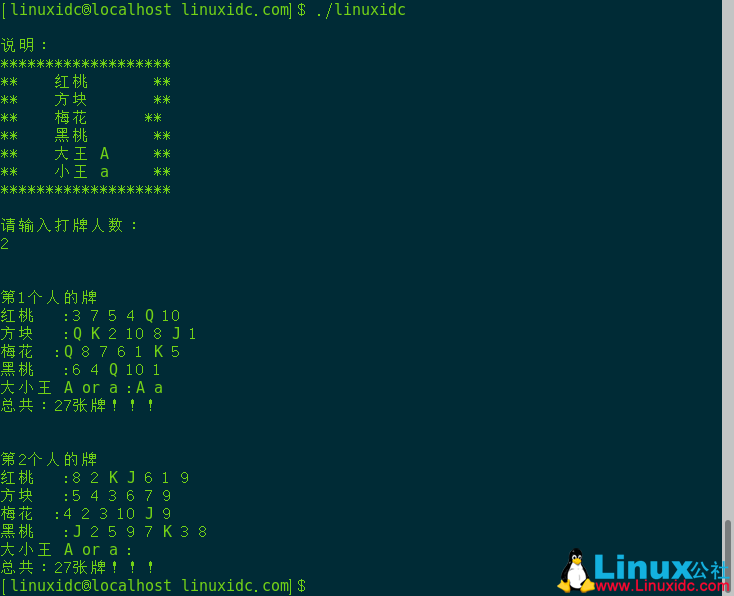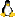• 请设计一个程序完成自动发牌工作。要求：黑桃用S (Spaces)表示，红桃用H (Hearts)表示，方块用D (Diamonds)表示，梅花用C (Clubs)表示。分析：要设置数组表现扑克牌要设置数组表现玩家要给扑克牌做特定标识，得到...

C语言自动发牌程序，供大家参考，具体内容如下

一副扑克有52张牌，打桥牌时应将牌分给4个人。请设计一个程序完成自动发牌的工作。要求：黑桃用S (Spaces)表示，红桃用H (Hearts)表示，方块用D (Diamonds)表示，梅花用C (Clubs)表示。

分析：

要设置数组表现扑克牌

要设置数组表现玩家

要给扑克牌做特定标识，得到结果后玩家要知道自己手中黑桃有哪些、方块有哪些

初步想法：

设置4个字符数组保存4种梅花牌，设置4个字符数组表示4名玩家分配到的牌

每张牌随机发给4名玩家，当玩家的持牌数达到13，不再分配给该名玩家牌

代码展示：

void mycode_13()

{

srand(unsigned(time(NULL)));

/*全部牌*/

char S = { '2', '3', '4', '5', '6', '7', '8', '9', 'T', 'J', 'Q', 'K', 'A' };

char H = { '2', '3', '4', '5', '6', '7', '8', '9', 'T', 'J', 'Q', 'K', 'A' };

char D = { '2', '3', '4', '5', '6', '7', '8', '9', 'T', 'J', 'Q', 'K', 'A' };

char C = { '2', '3', '4', '5', '6', '7', '8', '9', 'T', 'J', 'Q', 'K', 'A' };

/*4个玩家*/

char player1, player2, player3, player4;

int p1 = 0, p2 = 0, p3 = 0, p4 = 0;

distribution(S, player1, player2, player3, player4, &p1, &p2, &p3, &p4);

distribution(H, player1, player2, player3, player4, &p1, &p2, &p3, &p4);

distribution(D, player1, player2, player3, player4, &p1, &p2, &p3, &p4);

distribution(C, player1, player2, player3, player4, &p1, &p2, &p3, &p4);

puts("运行结束");

for (int i = 0; i < 13; i++)

printf("%c ", player1[i]);

putchar('\n');

for (int i = 0; i < 13; i++)

printf("%c ", player2[i]);

putchar('\n');

for (int i = 0; i < 13; i++)

printf("%c ", player3[i]);

putchar('\n');

for (int i = 0; i < 13; i++)

printf("%c ", player4[i]);

}

void distribution(char * S_H_D_C, char * player1, char * player2, char * player3, char * player4, int *p1, int *p2, int *p3, int *p4)

{

static int h = 1;

int r;

int a = *p1, b = *p2, c = *p3, d = *p4;

for (int i = 0; i < 13; i++)

{

r = (rand() % 4) + 1;

while ((r == 1 && (*p1) == 13) || (r == 2 && (*p2) == 13) || (r == 3 && (*p3) == 13) || (r == 4 && (*p4) == 13))

r = (rand() % 4) + 1;

switch (r)

{

case 1:

player1[(*p1)++] = S_H_D_C[i];

break;

case 2:

player2[(*p2)++] = S_H_D_C[i];

break;

case 3:

player3[(*p3)++] = S_H_D_C[i];

break;

case 4:

player4[(*p4)++] = S_H_D_C[i];

break;

default:

break;

}

}

switch (h++)

{

case 1:

printf("黑桃：\n");

break;

case 2:

printf("红桃：\n");

break;

case 3:

printf("方块：\n");

break;

case 4:

printf("梅花：\n");

break;

}

printf("Player1:");

for (int i = a; i < (*p1); i++)

printf("%c ", player1[i]);

putchar('\n');

printf("Player2:");

for (int i = b; i < (*p2); i++)

printf("%c ", player2[i]);

putchar('\n');

printf("Player3:");

for (int i = c; i < (*p3); i++)

printf("%c ", player3[i]);

putchar('\n');

printf("Player4:");

for (int i = d; i < (*p4); i++)

printf("%c ", player4[i]);

putchar('\n');

}

以下代码保证了当某个人得到13张牌后不在得牌

r = (rand() % 4) + 1;

while ((r == 1 && (*p1) == 13) || (r == 2 && (*p2) == 13) || (r == 3 && (*p3) == 13) || (r == 4 && (*p4) == 13))

r = (rand() % 4) + 1;

以上就是本文的全部内容，希望对大家的学习有所帮助，也希望大家多多支持脚本之家。

展开全文• 请设计一个程序完成自动发牌工作。要求：黑桃用S (Spaces)表示，红桃用H (Hearts)表示，方块用D (Diamonds)表示，梅花用C (Clubs)表示。分析：要设置数组表现扑克牌要设置数组表现玩家要给扑克牌做特定标识，得到...

C语言自动发牌程序，供大家参考，具体内容如下：

一副扑克有52张牌，打桥牌时应将牌分给4个人。请设计一个程序完成自动发牌的工作。要求：黑桃用S (Spaces)表示，红桃用H (Hearts)表示，方块用D (Diamonds)表示，梅花用C (Clubs)表示。

分析：

要设置数组表现扑克牌

要设置数组表现玩家

要给扑克牌做特定标识，得到结果后玩家要知道自己手中黑桃有哪些、方块有哪些

初步想法：

设置4个字符数组保存4种梅花牌，设置4个字符数组表示4名玩家分配到的牌

每张牌随机发给4名玩家，当玩家的持牌数达到13，不再分配给该名玩家牌

代码展示：void mycode_13()

{

srand(unsigned(time(NULL)));/*全部牌*/char S = { '2', '3', '4', '5', '6', '7', '8', '9', 'T', 'J', 'Q', 'K', 'A' };char H = { '2', '3', '4', '5', '6', '7', '8', '9', 'T', 'J', 'Q', 'K', 'A' };char D = { '2', '3', '4', '5', '6', '7', '8', '9', 'T', 'J', 'Q', 'K', 'A' };char C = { '2', '3', '4', '5', '6', '7', '8', '9', 'T', 'J', 'Q', 'K', 'A' };/*4个玩家*/char player1, player2, player3, player4;int p1 = 0, p2 = 0, p3 = 0, p4 = 0;

distribution(S, player1, player2, player3, player4, &p1, &p2, &p3, &p4);

distribution(H, player1, player2, player3, player4, &p1, &p2, &p3, &p4);

distribution(D, player1, player2, player3, player4, &p1, &p2, &p3, &p4);

distribution(C, player1, player2, player3, player4, &p1, &p2, &p3, &p4);

puts("运行结束");for (int i = 0; i

printf("%c ", player1[i]);

putchar('\n');for (int i = 0; i

printf("%c ", player2[i]);

putchar('\n');for (int i = 0; i

printf("%c ", player3[i]);

putchar('\n');for (int i = 0; i

printf("%c ", player4[i]);

}void distribution(char * S_H_D_C, char * player1, char * player2, char * player3, char * player4, int *p1, int *p2, int *p3, int *p4)

{static int h = 1;int r;int a = *p1, b = *p2, c = *p3, d = *p4;for (int i = 0; i

{

r = (rand() % 4) + 1;while ((r == 1 && (*p1) == 13) || (r == 2 && (*p2) == 13) || (r == 3 && (*p3) == 13) || (r == 4 && (*p4) == 13))

r = (rand() % 4) + 1;switch (r)

{case 1:

player1[(*p1)++] = S_H_D_C[i];  break;case 2:

player2[(*p2)++] = S_H_D_C[i];  break;case 3:

player3[(*p3)++] = S_H_D_C[i];  break;case 4:

player4[(*p4)++] = S_H_D_C[i];  break;default:  break;

}

}switch (h++)

{case 1:

printf("黑桃：\n");  break;case 2:

printf("红桃：\n");  break;case 3:

printf("方块：\n");  break;case 4:

printf("梅花：\n");  break;

}

printf("Player1:");for (int i = a; i

printf("%c ", player1[i]);

putchar('\n');

printf("Player2:");for (int i = b; i

printf("%c ", player2[i]);

putchar('\n');

printf("Player3:");for (int i = c; i

printf("%c ", player3[i]);

putchar('\n');

printf("Player4:");for (int i = d; i

printf("%c ", player4[i]);

putchar('\n');

}

以下代码保证了当某个人得到13张牌后不在得牌：r = (rand() % 4) + 1;while ((r == 1 && (*p1) == 13) || (r == 2 && (*p2) == 13) || (r == 3 && (*p3) == 13) || (r == 4 && (*p4) == 13))

r = (rand() % 4) + 1;

以上就是本文的全部内容，希望对大家的学习有所帮助，也希望大家多多支持我。

展开全文• 请设计一个程序完成自动发牌工作。要求：黑桃用S (Spaces)表示，红桃用H (Hearts)表示，方块用D (Diamonds)表示，梅花用C (Clubs)表示。 问题分析 按照打桥牌的规定，每人应当有13张牌。在人工发牌时，先进行洗...

问题描述

一副扑克有52张牌，打桥牌时应将牌分给4个人。请设计一个程序完成自动发牌的工作。要求：黑桃用S (Spaces)表示，红桃用H (Hearts)表示，方块用D (Diamonds)表示，梅花用C (Clubs)表示。

问题分析

按照打桥牌的规定，每人应当有13张牌。在人工发牌时，先进行洗牌，然后将洗好的牌按一定的顺序发给每一个人。为了便于计算机模拟，可将人工方式的发牌过程加以修改：先确定好发牌顺序：1、2、3、4；将52张牌顺序编号：黑桃2对应数字0，红桃2对应数字1，方块2对应数字2，梅花2对应数字3，黑桃3对应数字4，红桃3对应数字5，……； 然后从52张牌中随机为每个人抽牌。

下面是完整的代码：

#include<stdlib.h>
#include<stdio.h>
int comp(const void *j, const void *i);
void p(int b[], char n[]);
int main(void)
{
static char n[]={'2', '3', '4', '5', '6', '7', '8', '9', 'T', 'J', 'Q', 'K', 'A'};
int a, b1, b2, b3, b4;
int b11=0, b22=0, b33=0, b44=0, t=1, m, flag, i;
while( t<=52 )  /*控制发52张牌*/
{
m=rand()%52;  /*产生0到51之间的随机数*/
for(flag=1,i=1; i<=t&&flag; i++)  /*查找新产生的随机数是否已经存在*/
if(m==a[i])
flag=0;  /*flag=1表示产生的是新的随机数，flag=0表示新产
生的随机数已经存在*/
if(flag)
{
a[t++]=m;  /*如果产生了新的随机数，则存入数组*/
/*根据t的模值，判断当前的牌应存入哪个数组中*/
if(t%4==0)
b1[b11++]=a[t-1];
else
if(t%4==1)
b2[b22++]=a[t-1];
else
if(t%4==2)
b3[b33++]=a[t-1];
else
if(t%4==3)
b4[b44++]=a[t-1];
}
}
qsort(b1, 13, sizeof(int), comp);  /*将每个人的牌进行排序*/
qsort(b2, 13, sizeof(int), comp);
qsort(b3, 13, sizeof(int), comp);
qsort(b4, 13, sizeof(int), comp);
p(b1, n);  /*分别打印每个人的牌*/
p(b2, n);
p(b3, n);
p(b4, n);
return 0;
}
void p(int b[], char n[])
{
int i;
printf("\n\006 ");  /*打印黑桃标记*/
for(i=0; i<13; i++)  /*将数组中的值转换为相应的花色*/
if(b[i]/13==0)  /*找到该花色对应的牌*/
printf("%c ", n[b[i]%13]);
printf("\n\003 ");  /*打印红桃标记*/
for(i=0; i<13; i++)
if((b[i]/13)==1)
printf("%c ", n[b[i]%13]);
printf("\n\004 ");  /*打印方块标记*/
for(i=0; i<13; i++)
if(b[i]/13==2)
printf("%c ", n[b[i]%13]);
printf("\n\005 ");  /*打印梅花标记*/
for(i=0; i<13; i++)
if(b[i]/13==3 || b[i]/13==4)
printf("%c ", n[b[i]%13]);
printf("\n");
}
int comp(const void *j, const void *i)  /*qsort调用的排序函数*/
{
return(*(int*)i-*(int*)j);
}

运行结果：

Q T 2  8  8 7 5 4  A T 9 7 3

A 9 8 5  K 6 2  Q T 9 6  J 6

K J A Q J T 7 K J 3 2 5 2

- 7 6 4 3 9 5 4 3  A  K Q 8 4

展开全文c语言
• 请设计一个程序完成自动发牌工作。要求：黑桃用S (Spaces)表示，红桃用H (Hearts)表示，方块用D (Diamonds)表示，梅花用C (Clubs)表示。问题分析按照打桥牌的规定，每人应当有13张牌。在人工发牌时，先进行洗牌，...

问题描述

一副扑克有52张牌，打桥牌时应将牌分给4个人。请设计一个程序完成自动发牌的工作。要求：黑桃用S (Spaces)表示，红桃用H (Hearts)表示，方块用D (Diamonds)表示，梅花用C (Clubs)表示。

问题分析

按照打桥牌的规定，每人应当有13张牌。在人工发牌时，先进行洗牌，然后将洗好的牌按一定的顺序发给每一个人。为了便于计算机模拟，可将人工方式的发牌过程加以修改：先确定好发牌顺序：1、2、3、4；将52张牌顺序编号：黑桃2对应数字0，红桃2对应数字1，方块2对应数字2，梅花2对应数字3，黑桃3对应数字4，红桃3对应数字5，……； 然后从52张牌中随机为每个人抽牌。

下面是完整的代码：

#include

#include

#include

int main()

{

int a;

int b;

int w = 0;

int wi,wj,wii;

int i,j,index,x=0,m;

for(i=0;i<54;i++)

a[i]=i+1;

srand((unsigned)time(0));

for(i=0;i<54;)

{

index=rand()%54;

if(a[index]!=0)

{

b[i]=a[index];

//printf("%d ",b[i]);

a[index]=0;

++i;

}

}

printf("\n说明：\n");

printf("*******************\n");

printf("**    红桃 %c    ",3);

printf("**\n");

printf("**    方块 %c    ",4);

printf("**\n");

printf("**    梅花 %c    ",5);

printf("**\n");

printf("**    黑桃 %c    ",6);

printf("**\n");

printf("**    大王 A    **\n**    小王 a    **\n");

printf("*******************\n");

printf("\n请输入打牌人数：\n");

scanf("%d",&w);

wi = 54/w;

wj = 54%w;

//printf("%d,%d",wi,wj);

for (j = 0;j < w;j++)

{

m = 0;

printf("\n\n第%d个人的牌\n",j+1);

wj --;

if (wj>=0)

wii = wi + 1;

else

wii = wi;

//红桃

printf("红桃 %c ",3);

printf(":");

for(i = j*wii;i

{

if (b[i]<=13&&b[i]>0)

{

m++;

if (b[i]==11)

printf("J ");

else if (b[i]==12)

printf("Q ");

else if (b[i]==13)

printf("K ");

else

printf("%d ",b[i]);

}

}

//方块

printf("\n方块 %c ",4);

printf(":");

for(i = j*wii;i

{

if (b[i]<=26&&b[i]>13)

{

m++;

b[i] = b[i]-13;

if (b[i]==11)

printf("J ");

else if (b[i]==12)

printf("Q ");

else if (b[i]==13)

printf("K ");

else

printf("%d ",b[i]);

}

}

//梅花

printf("\n梅花 %c ",5);

printf(":");

for(i = j*wii;i

{

if (b[i]<=39&&b[i]>26)

{

m++;

b[i] = b[i]-26;

if (b[i]==11)

printf("J ");

else if (b[i]==12)

printf("Q ");

else if (b[i]==13)

printf("K ");

else

printf("%d ",b[i]);

}

}

//黑桃

printf("\n黑桃 %c ",6);

printf(":");

for(i = j*wii;i

{

if (b[i]<=52&&b[i]>39)

{

m++;

b[i] = b[i]-39;

if (b[i]==11)

printf("J ");

else if (b[i]==12)

printf("Q ");

else if (b[i]==13)

printf("K ");

else

printf("%d ",b[i]);

}

}

//大小王

printf("\n大小王 A or a :");

for(i = j*wii;i<=(j+1)*wii;i++)

{

if (b[i]==53)

{

m++;

printf("A ");

}

if (b[i]==54)

{

m++;

printf("a ");

}

}

printf("\n总共：%d张牌！！！\n",m);

}

}

2个人分牌的测试结果

[linuxidc@localhost linuxidc.com]\$ ./linuxidc

说明：

*******************

**    红桃     **

**    方块     **

**    梅花      **

**    黑桃     **

**    大王 A    **

**    小王 a    **

*******************

请输入打牌人数：

2

第1个人的牌

红桃 :3 7 5 4 Q 10

方块 :Q K 2 10 8 J 1

梅花  :Q 8 7 6 1 K 5

黑桃 :6 4 Q 10 1

大小王 A or a :A a

总共：27张牌！！！

第2个人的牌

红桃 :8 2 K J 6 1 9

方块 :5 4 3 6 7 9

梅花  :4 2 3 10 J 9

黑桃 :J 2 5 9 7 K 3 8

大小王 A or a :

总共：27张牌！！！展开全文c语言自动打牌
• C语言每日一练——第64天：自动发牌程序

万次阅读 多人点赞 2021-12-07 15:49:28
请设计一个程序完成自动发牌工作。要求：黑桃用S (Spaces)表示，红桃用H (Hearts)表示，方块用D (Diamonds)表示，梅花用C (Clubs)表示。 问题分析 个人思路: 这题实现起来还是比较简单的，只需定义两个多维的字符...c语言
• 一、魔术师发牌问题1.问题描述魔术师利用一副牌中的13张黑牌，预先将他们排好后叠放在一起，牌面朝下。对观众说：“我不看牌，只数数就可以猜到每张牌是什么。”魔术师把最上面的那张牌数为1，把他翻过来正好是黑桃A...
• 主要分为4步，买牌，洗牌，发牌，看牌，采用junit单元测试，需要导入junit的jar包。 具体步骤： 1.准备工作 //1.定义一个双列集合,键：表示牌的编号。值：表示牌具体的值 Map<Integer,String> pokers=new ...java
• 虽然它在物理组成上也可以是星型结构连接，但在逻辑上仍然以环的方式进行工作。其通信传输介质可以是无屏蔽双绞线、屏蔽双绞线和光纤等。 令牌环网的媒体接入控制机制采用的是分布式控制模式的循环方法。在令牌环网...网络
• 原标题：斗地主用机器人发牌，乐高这么玩你想试试吗？在很多朋友的手机里面，都会有一款非常经典的游戏，那就是斗地主。睡着斗一会儿，公交车上斗一会儿，反正有零碎时间比较无聊了，就可以玩一会儿。但是，如果好...
• 经常有朋友问我，为什么看着前端和系统功能都一样，只有LOGO不同。其实只是系统的品牌名字不一样！这个对于我们技术来说就是做一个前端的logo设计，logo需要的尺寸250*40的尺寸，png格式的图片就OK。作者：LAU768 ...erp 数据库 java github
• 一副:54张从0开始排序:0-12表示黑桃 A 1,2,3,... 10,J,Q,K13-25表示红桃 A 1,2,3,... 10,J,Q,K26-38表示草花 A 1,2,3,... 10,J,Q,K39-51表示方块 A 1,2,3,... 10,J,Q,K52,53表示大小王#include void shuffle(char ...
• 发牌 2.判断牌型 3.比较牌的大小 4.开始游戏 5.总结 说明： 制作的德州扑克仅供参考，提供源码，源码可运行 可满足基本操作，另外还有一些小功能，可先自行研究 和真正的德州扑克游戏相比，九牛一毛，可...
• 汪峰善于计算发牌概率 据知情人透露，汪峰玩德州扑克走的是技术流，他能大概算出每一次发牌的概率，还有通过牌桌上其他玩家的表情动作推测出对方的底牌，这让汪峰一直是比赛中的大赢家。 仅仅是大赢家还不够，在...
• 什么是Ajax?Ajax是“Asynchronous JavaScript and XML”的缩写。他是指一种创建交互式网页应用的网页 开发技术。沟通客户端与服务器，可以在【不必刷新整个浏览器】的情况下，与服务器进行异步通讯的技术。Ajax的...
• 5大新锐品牌自播GMV创历史新高，背后的逻辑是什么？ 2021-07-31 15:44 从花西子的中国东方美妆护肤，元气森林的0糖0脂，品牌市场竞争已不会再限于传统式的方式、价钱、商品，只是进入了一场高维战事，品牌、顾客...数据库
• 黄波将一整副扑克放在左手，五根手指稍微转动了一下，一副平均分成了两半。随后，他前三根手指拿一半，后两根手指控制另一半，开始用一只手洗。左边一张，右边一张，扑克一张一张分别从这两半中均匀地叠在了...
• } // 发牌顺序计算 void Magician(linklist head) { linklist p; int j; int Countnumber = 2; p = head; p->data = 1; while(1) { for(j=0; j ; j++) { p = p->next; if(p->data != 0) { p->next; j--; } } if(p->...算法 c++ 链表 数据结构
• 【Java数据结构】初识集合框架——List的使用（附加自动发牌案例）泛型什么是泛型泛型的分类泛型的定义简单演示泛型背后作用时期和背后的简单原理泛型类的使用泛型总结包装类基本数据类型和包装类直接的对应关系包装...java 链表 数据结构
• 30 扑克游戏 小明同学想要做一个扑克游戏， 他在列表里面存了 1 到 13 的数 字，分别代表 A、2、3……J、Q、K，程序运行后会随机从列表里面抽 取 3 个数字，并将数值存到列表【 ch】中，但是他遇到了一个问题，...Scratch游戏 Scratch编程
• 企业管理5h是什么意思

千次阅读 2020-12-30 09:17:36
企业人才培养工作要注意哪些问题企业对于人力资源开发工作的重视源于人才对于企业重要性的提升，这直接导致了现代企业越来越重视培养人才的工作，要做好企业人才培养和企业内优秀的团队建设工作主要有一下5各方面的...
• 记得当初在写一个js版本的斗地主程序的时候，我让朋友用ps给我制作了54张扑克(是54张吗？)后来想换的大小，又不好再让人家做，当时是左右为难，今天在国外的网站上看到了一篇css制作扑克的文章，其效果比ps的...
• 先不要脾气，只谈正题，谈完了再让你脾气。他就笑了。 第二部应该研究的书是什么呢？就是黄石公传给张良的《素书》，这一部书很难说确是伪书，但它也的确是中国文化的结晶。对于为人处世及认识人物的道理，有很...
• 然后过滤器会检查请求对象，并决定将该请求转发给链中的下一个组件，或者中止该请求并直接向客户机回一个响应。如果请求被 转发了，它将被传递给链中的下一个资源（另一个过滤器、servlet 或 JSP 页面）。在这个...什么是java过滤器
• 同时上市的公司还要做很多上市前工作：审计、找保荐人、找发行承销商等准备，每项都是要钱的，随便一个上亿资本的公司，光审计费用就要好几万、好几十万甚至上千万的花销。保荐人的工资年薪就200万起，这些钱其实也...
• 总结 我向来佩服华为外包工的魄力和胆识，不管你出于什么原因选择在别人看来‘不体面’的工作，你都在用实际行动证明：敢想敢干才是修仙逆袭的终极法门！ 总之加油吧，华为外包工！ 技术交流群 最近有很多人问，有...java 大数据 人工智能 编程语言
• 大家好，现在淘宝店铺的运营工作是非常的重要，但是很多人不知道这个运营每天需要做什么，对于工作的重心不知道是做什么的，下面就来介绍下作为一名运营工作者，每天需要做那些工作? 1、浏览店铺数据，及时分析......